# CLASS 12 MATHS CHAPTER 4-DETERMINANTS

### Solve The Following Questions.

Evaluate the following determinants in Exercise 1 and 2.

Question1.

Solution :
= 2(-1) – 4(-5) = -2 + 20 = 18

Question2. (i)

(ii)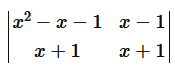Solution :
(i)

= (cosθ)(cosθ) – (-sinθ) (sinθ)= cos2 θ + sin2 θ= 1

(ii)= (x2 − x + 1)(x + 1) − (x − 1)(x + 1)

x3 − x2 + x + x2 − x + 1 − (x2 − 1)

x3 + 1 − x2 + 1

x3 − x2 + 2

#### Question3. If A =  then show that |2A| = 4|A|

Solution :
Given: A =

then 2A = 2 x

Hence, proved.

Question4. If A =  then show that 3|A| = 27|A|

Solution :
Given: A =  then 3A =3

It can be observed that in the first column, two entries are zero. Thus, we expand along the first column (C1) for easier calculation.

Hence, proved.

Question5. Evaluate the determinants:

(i)

(ii)

(iii)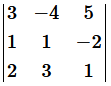(iv)

Solution :
Evaluate the determinants:

(i) Given:

It can be observed that in the second row, two entries are zero. Thus, we expand along the second row for easier calculation.

=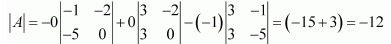(ii) Given:

By expanding along the first row, we have:

=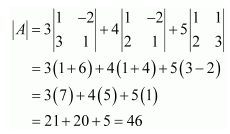(iii) Given:Expanding along first row,

=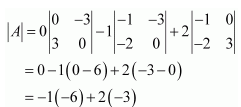= 0 + 6 – 6 = 0

(iv) Given:

Expanding along first row,

=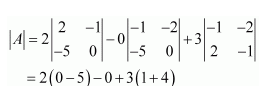= -10 + 15 = 5

Question6. If A =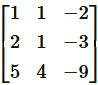find |A|

Solution :
Given:     A =Expanding along first row,

=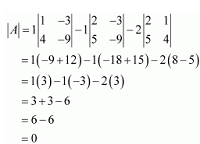Question7. Find the value of x if:

(i)

(ii)

Solution :
(i) Given:

⇒ 2 x 1 – 5 x 4 = 2x * x – 6 x 4

⇒ 2 – 20 = 2x2 – 24

⇒ 2x= 6

⇒  x= 3

⇒ x = ± √3

(ii)

⇒ 2 x 5 – 4 x 3 = x * 5 – 2x – 3

⇒10 – 12 = 5x – 6x

⇒ – 2 = -x

⇒ x = 2

Question8. If  then x is equal to:

(A) 6

(B) ± 6

(C) – 6

(D) 0

Solution :
Given:

⇒x * x – 18 x 2 = 6 x 6 – 18 x 2

⇒x2 – 36 = 36 – 36

⇒x2 – 36 = 0

⇒x = ± 6

Therefore, option (B) is correct.

### Solve The Following Questions.

Using the properties of determinants and without expanding in Exercise 1 to 5, prove that:

Question1.

Solution :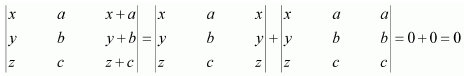[Here, two columns of the determinants are identical]

Question 2.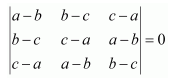Solution :
On Operating

Question3.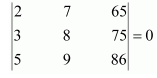Solution :

Question4.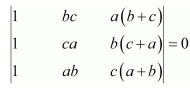Solution :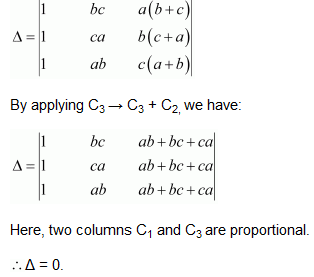Question5.

Solution :

=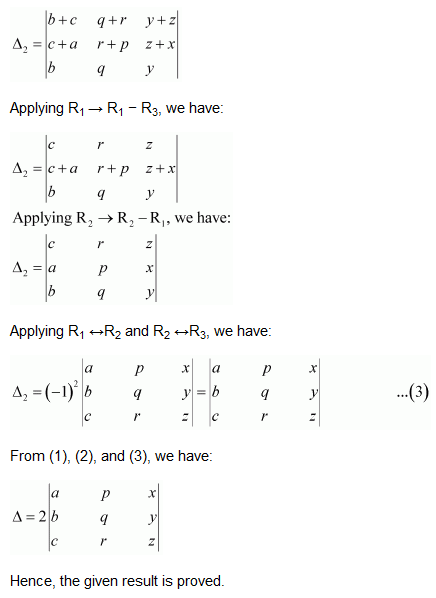Question6.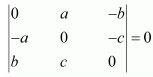Solution :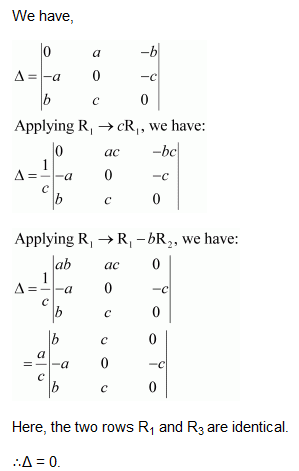Question7.

Solution :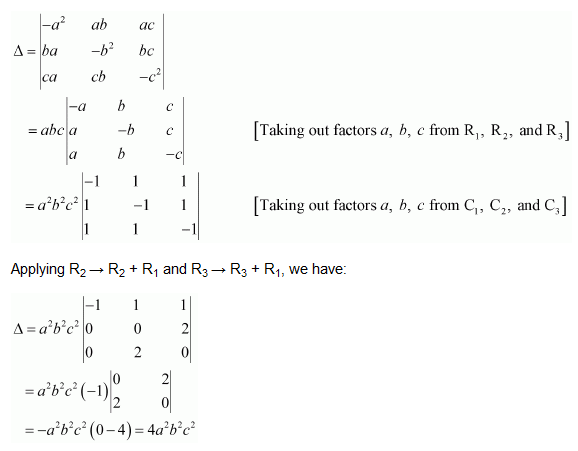Question8.

Solution :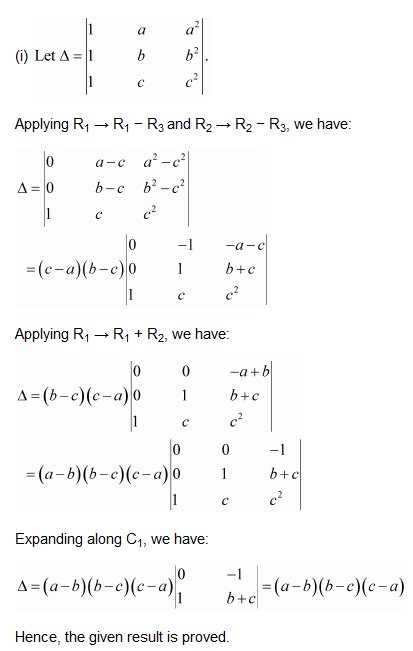=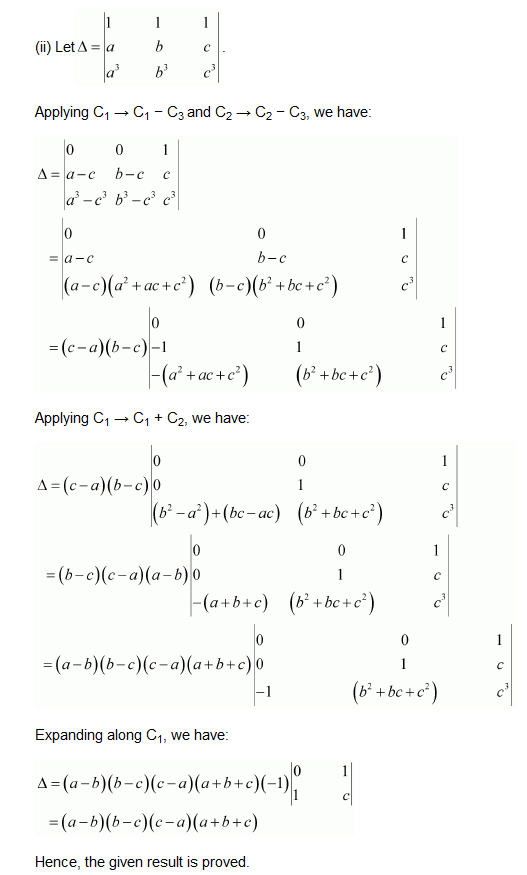Question9.

Solution :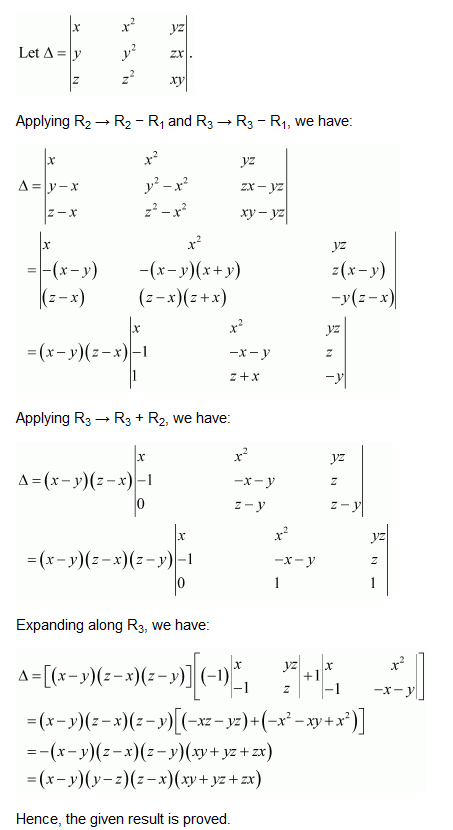Question10. (i)

(ii)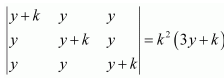Solution :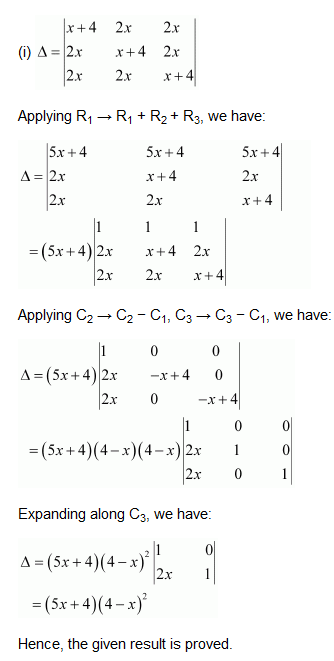Question11. (i)

(ii)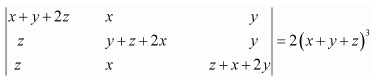Solution :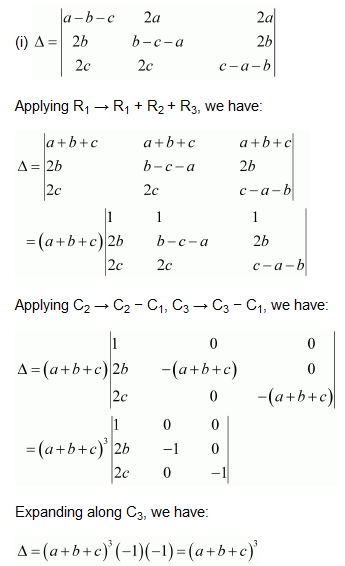Question12.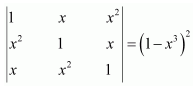Solution :

Question13.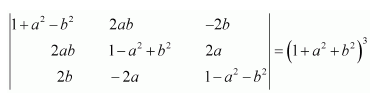Solution :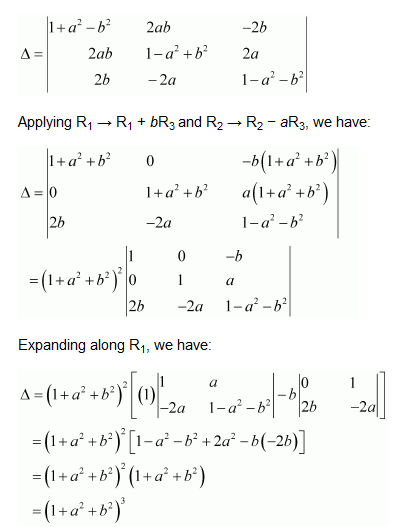Question14.

Solution :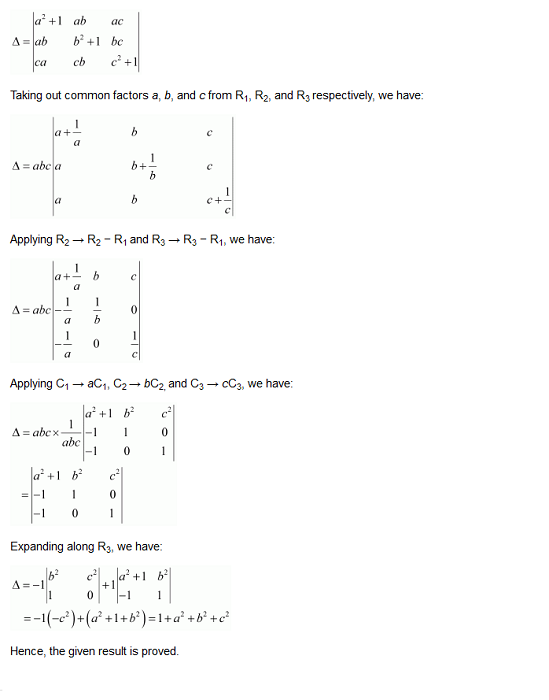Question15. Let A be a square matrix of order 3 x 3, then k |A| is equal to:

(A) k |A|

(B) k2 |A|

(C) k3 |A|

(D) 3k |A|

Solution :

Therefore, option (C) is correct.

### Solve The Following Questions.

Question1. Find the area of the triangle with vertices at the points given in each of the following:

(i) (1, 0), (6, 0), (4, 3)

(ii) (2, 7), (1, 1), (10, 8)

(iii) (−2, −3), (3, 2), (−1, −8)

Solution :

(i) The area of the triangle with vertices (1, 0), (6, 0), (4, 3) is given by the relation,

=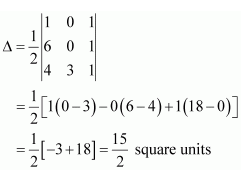(ii) The area of the triangle with vertices (2, 7), (1, 1), (10, 8) is given by the relation,

=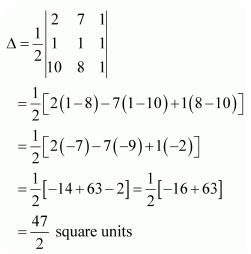(iii) The area of the triangle with vertices (−2, −3), (3, 2), (−1, −8)

=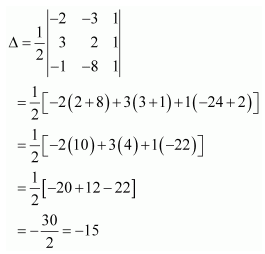Question2. Show that the points A(a,b + c), B(b, c + a), C(c, a+b) are collinear.

Solution :

Therefore, points A, B and C are collinear.

Question3. Find values of k if area of triangle is 4 sq. units and vertices are:

(i) (k, 0), (4, 0), (0, 2)

(ii) (−2, 0), (0, 4), (0, k)

Solution :

We know that the area of a triangle whose vertices are (x1y1), (x2y2), and

(x3y3) is the absolute value of the determinant (Δ), where

When −k + 4 = − 4, k = 8.

When −k + 4 = 4, k = 0.

Hence, k = 0, 8.

(ii) The area of the triangle with vertices (−2, 0), (0, 4), (0, k) is given by the relation,

k − 4 = ± 4

When k − 4 = − 4, k = 0.

When k − 4 = 4, k = 8.

Hence, k = 0, 8.

Question4. (i) Find the equation of the line joining (1, 2) and (3, 6) using determinants.

(ii) Find the equation of the line joining (3, 1) and (9, 3) using determinants.

Solution :
(i) Let P(x, y) be any point on the line joining the points (1, 2) and (3, 6).

Then, Area of triangle that could be formed by these points is zero.

Hence, the equation of the line joining the given points is y = 2x.

(ii) Let P (xy) be any point on the line joining points A (3, 1) and

B (9, 3). Then, the points A, B, and P are collinear. Therefore, the area of triangle ABP will be zero.

Hence, the equation of the line joining the given points is x − 3y = 0.

Question5. If area of triangle is 35 square units with vertices (2, −6), (5, 4), and (k, 4). Then k is

(A). 12

(B). −2

(C). −12, −2

(D). 12, −2

Solution :

The area of the triangle with vertices (2, −6), (5, 4), and (k, 4) is given by the relation,

It is given that the area of the triangle is ±35.

Therefore, we have:

⇒ 25 – 5k = ± 35

⇒ 5(5 – k) = ± 35

⇒ 5 – k = ± 7

When 5 − k = −7, k = 5 + 7 = 12.

When 5 − k = 7, k = 5 − 7 = −2.

Hence, k = 12, −2.

Therefore, option (D) is correct.

### Solve The Following Questions.

Question1. Write minors and cofactors of the elements of the following determinants:

(i)

(ii)

Solution :
(i) Let

Minor of element aij is Mij.

∴M11 = minor of element a11 = 3

M12 = minor of element a12 = 0

M21 = minor of element a21 = −4

M22 = minor of element a22 = 2

Cofactor of aij is Aij = (−1)i + j Mij.

∴A11 = (−1)1+1 M11 = (−1)2 (3) = 3

A12 = (−1)1+2 M12 = (−1)3 (0) = 0

A21 = (−1)2+1 M21 = (−1)3 (−4) = 4

A22 = (−1)2+2 M22 = (−1)4 (2) = 2

(ii) Let

Minor of element aij is Mij.

∴M11 = minor of element a11 d

M12 = minor of element a12 b

M21 = minor of element a21 c

M22 = minor of element a22 a

Cofactor of aij is Aij = (−1)i + j Mij.

∴A11 = (−1)1+1 M11 = (−1)2 (d) = d

A12 = (−1)1+2 M12 = (−1)3 (b) = −b

A21 = (−1)2+1 M21 = (−1)3 (c) = −c

A22 = (−1)2+2 M22 = (−1)4 (a) = a

Question2. Write minors and cofactors of the elements of the following determinants:

Solution :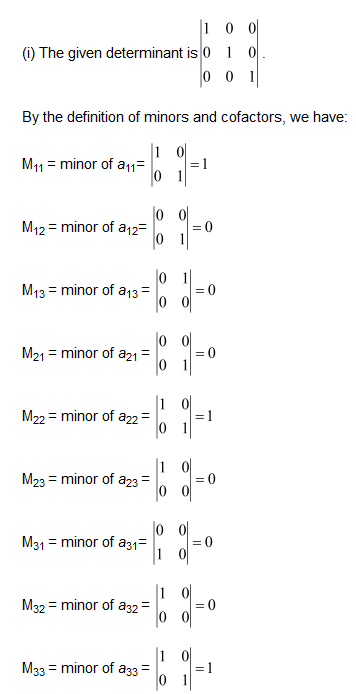A11 = cofactor of a11= (−1)1+1 M11 = 1

A12 = cofactor of a12 = (−1)1+2 M12 = 0

A13 = cofactor of a13 = (−1)1+3 M13 = 0

A21 = cofactor of a21 = (−1)2+1 M21 = 0

A22 = cofactor of a22 = (−1)2+2 M22 = 1

A23 = cofactor of a23 = (−1)2+3 M23 = 0

A31 = cofactor of a31 = (−1)3+1 M31 = 0

A32 = cofactor of a32 = (−1)3+2 M32 = 0

A33 = cofactor of a33 = (−1)3+3 M33 = 1

A11 = cofactor of a11= (−1)1+1 M11 = 11

A12 = cofactor of a12 = (−1)1+2 M12 = −6

A13 = cofactor of a13 = (−1)1+3 M13 = 3

A21 = cofactor of a21 = (−1)2+1 M21 = 4

A22 = cofactor of a22 = (−1)2+2 M22 = 2

A23 = cofactor of a23 = (−1)2+3 M23 = −1

A31 = cofactor of a31 = (−1)3+1 M31 = −20

A32 = cofactor of a32 = (−1)3+2 M32 = 13

A33 = cofactor of a33 = (−1)3+3 M33 = 5

Question3. Using cofactors of elements of second row, evaluate: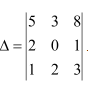Solution :

We know that Δ is equal to the sum of the product of the elements of the second row with their corresponding cofactors.

∴Δ = a21A21 + a22A22 + a23A23 = 2(7) + 0(7) + 1(−7) = 14 − 7 = 7

Question4. Using cofactors of elements of third column, evaluate:

Solution :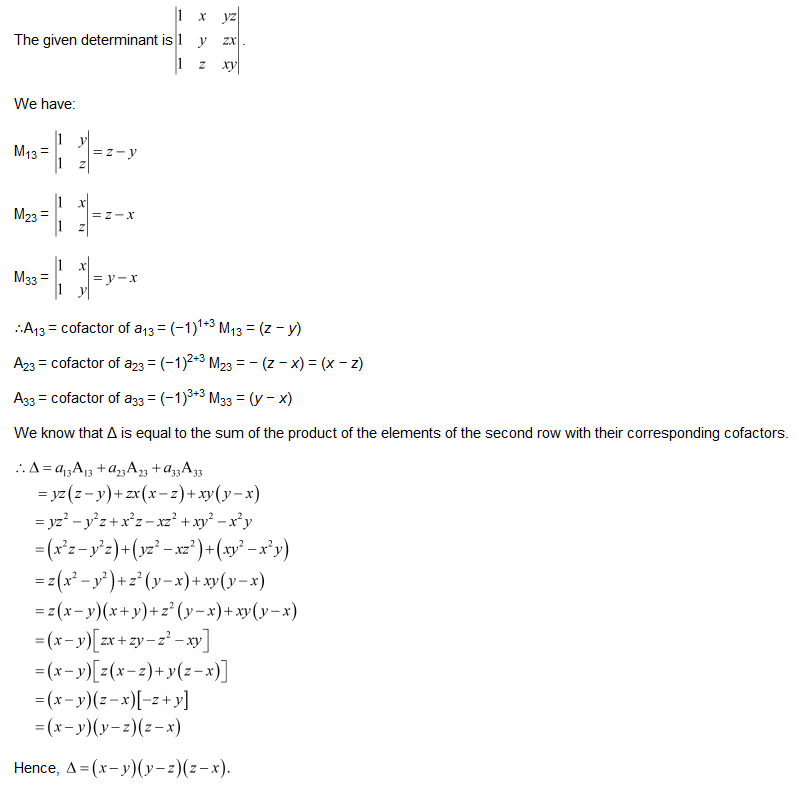Question5. If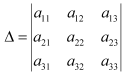and Aij is Cofactors of aij, then value of Δ is given by

Solution :

We know that:

Δ = Sum of the product of the elements of a column (or a row) with their corresponding cofactors

∴Δ = a11A11 + a21A21 + a31A31

Hence, the value of Δ is given by the expression given in alternative D.
Option (D) is correct.

### Solve The Following Questions.

Find adjoint of each of the matrices in Exercise 1 and 2.

Question1.

Solution :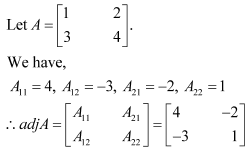Question2.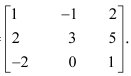Solution :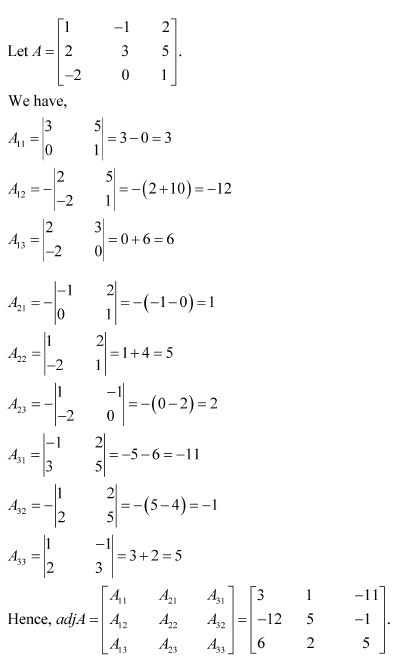Question3.

Solution :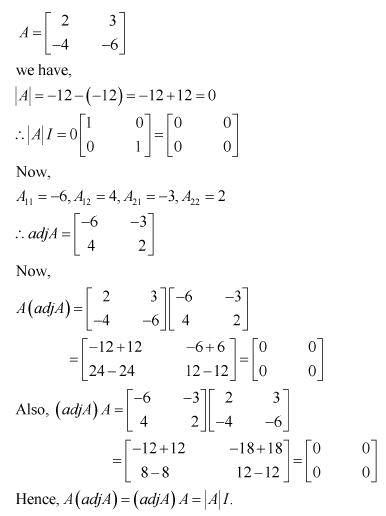Question4.

Solution :
Let A =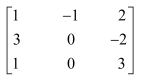Find the inverse of the matrix (if it exists) given in Exercise 5 to 11.

Question5.

Solution :

Question6.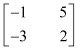Solution :

Question7.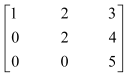Solution :

Question8.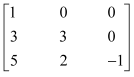Solution :

Question9.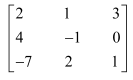Solution :

Question10.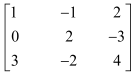Solution :
Let A =Question11.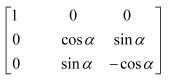Solution :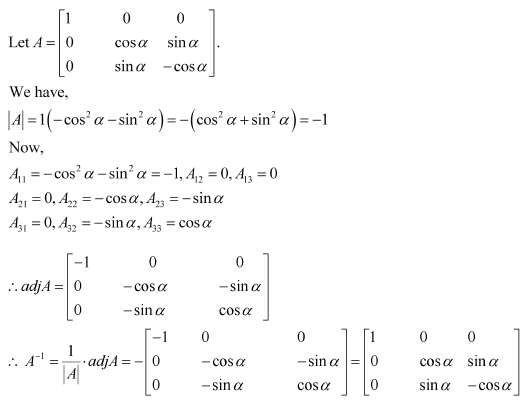Question12. Let

Solution :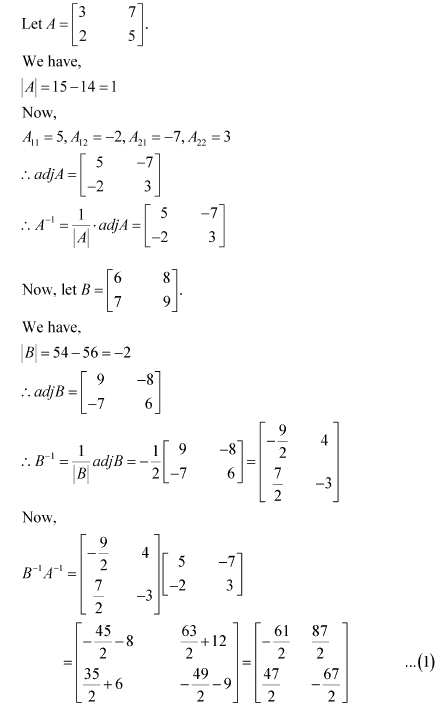Question13. If A =, show that A2 – 5A + 7I = 0. Hence find A-1

Solution :

Question14. For the matrix A = find numbers a and b such that A2 + aA + bI O.

Solution :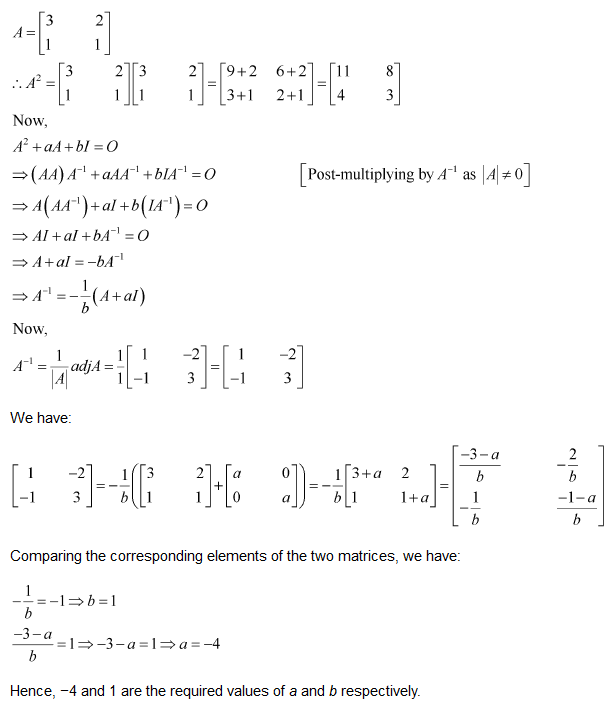Question15. For the matrix A =, show that A3 − 6A2 + 5A + 11 I = O. Hence, find A−1.

Solution :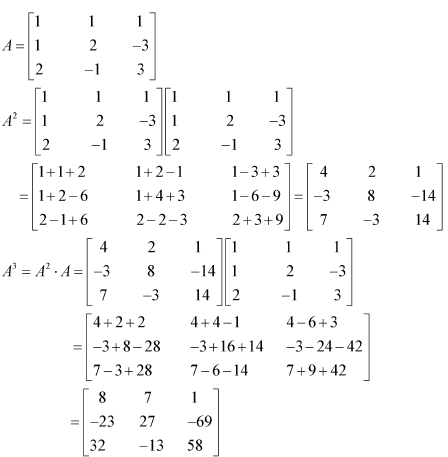Question16. If A =, verify that A3 − 6A2 + 9A − 4I = O and hence find A−1

Solution :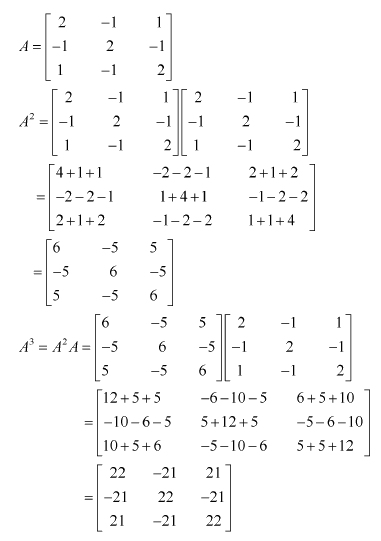Question17. Let A be a non-singular matrix of order 3 x 3. Then |adjA| is equal to:

(A) |A|

(B) |A|2

(C) |A|3

(D) 3|A|

Solution :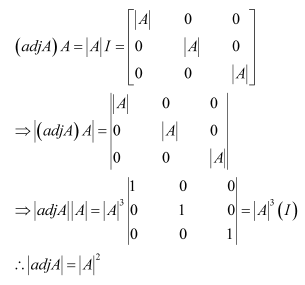Therefore, option (B) is correct.

Question18. If A is an invertible matrix of order 2, then det (A−1) is equal to:

(A) det A

(B) 1/det A

(C) 1

(D) 0

Solution :

Therefore, option (B) is correct.

### Solve The Following Questions.

Examine the consistency of the system of equations in Exercises 1 to 3.

Question1.

+ 2= 2

2x + 3= 3

Solution :
Matrix form of given equations is AX = B

∴ A is non-singular.

Therefore, A−1 exists.

Hence, the given system of equations is consistent.

Question2.

2− y = 5

x + = 4

Solution :
Matrix form of given equations is AX = B

∴ A is non-singular.

Therefore, A−1 exists.

Hence, the given system of equations is consistent.

Question3.

x + 3y = 5

2x + 6y = 8

Solution :
Matrix form of given equations is AX = B

∴ A is a singular matrix.

Thus, the solution of the given system of equations does not exist. Hence, the system of equations is inconsistent.

Examine the consistency of the system of equations in Exercises 4 to 6.

Question4.

x + y z = 1

2x + 3y + 2z = 2

ax + ay + 2az = 4

Solution :
Matrix form of given equations is AX = B

∴ A is non-singular.

Therefore, A−1 exists.

Hence, the given system of equations is consistent.

Question5.

3x − y − 2z = 2

2y − z = −1

3x − 5y = 3

Solution :
Matrix form of given equations is AX = B

Thus, the solution of the given system of equations does not exist. Hence, the system of equations is inconsistent.

Question6.

5x − y + 4z = 5

2x + 3y + 5z = 2

5x − 2y + 6z = −1

Solution :
Matrix form of given equations is AX = B

∴ A is non-singular.

Therefore, A−1 exists.

Hence, the given system of equations is consistent.

Solve the system of linear equations, using matrix method, in Exercise 7 to 10.

Question7.

5x + 2y =4

7x + 3y = 5

Solution :
Matrix form of given equations is AX = B

Question8.

2x – y = – 2

3x + 4y = 3

Solution :
Matrix form of given equations is AX = B

Question9.

4x – 3y = 3

3x – 5y = 7

Solution :
Matrix form of given equations is AX = B

Question10.

5x + 2y = 3

3x + 2y = 5

Solution :
Matrix form of given equations is AX = B

Thus, A is non-singular. Therefore, its inverse exists.

Solve the system of linear equations, using matrix method, in Exercise 11 to 14.

Question11.

Solution :
Matrix form of given equations is AX = B

Question12.

x − y + z = 4

2x + y − 3z = 0

x + y + z = 2

Solution :
Matrix form of given equations is AX = B

Question13.

2x + 3y + 3z = 5

x − 2y + z = −4

3x − y − 2z = 3

Solution :
Matrix form of given equations is AX = B

Question14.

x − y + 2z = 7

3x + 4y − 5z = −5

2x − y + 3z = 12

Solution :
Matrix form of given equations is AX = B

Question15. If A =  find A−1. Using A−1 solve the system of equations

Solution :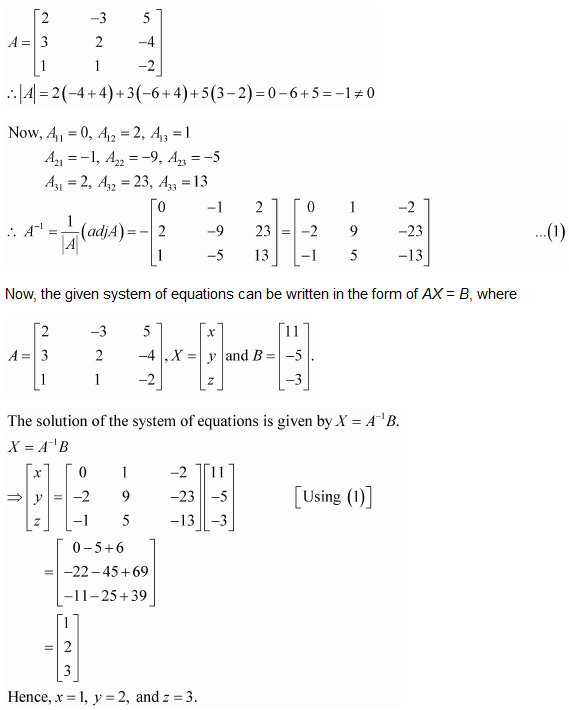Question16. The cost of 4 kg onion, 3 kg wheat and 2 kg rice is ` 60. The cost of 2 kg onion, 4 kg wheat and 2 kg rice is ` 90. The cost of 6 kg onion, 2 k wheat and 3 kg rice is ` 70. Find cost of each item per kg by matrix method.

Solution :

Let the cost of onions, wheat, and rice per kg be Rs x, Rs y,and Rs z respectively.

Then, the given situation can be represented by a system of equations as:

4x + 3y + 2z = 60

2x + 4y + 6z = 90

6x + 2y + 3z = 70

This system of equations can be written in the form of AX = B, where

Hence, the cost of onions is Rs 5 per kg, the cost of wheat is Rs 8 per kg, and the cost of rice is Rs 8 per kg.

### Solve The Following Questions.

Question1. Prove that the determinant is independent of θ.

Solution :

Hence, Δ is independent of θ.

Question2. Without expanding the determinants, prove that:

Solution :

Question3. Evaluate:

Solution :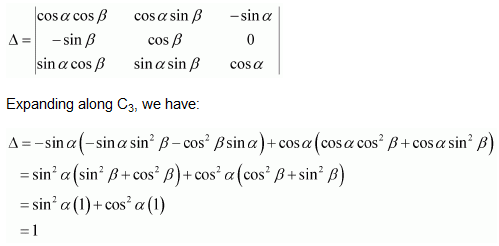Question4. If a,b and c are real numbers and

Show that either a + b + c = 0 or a = b = c.

Solution :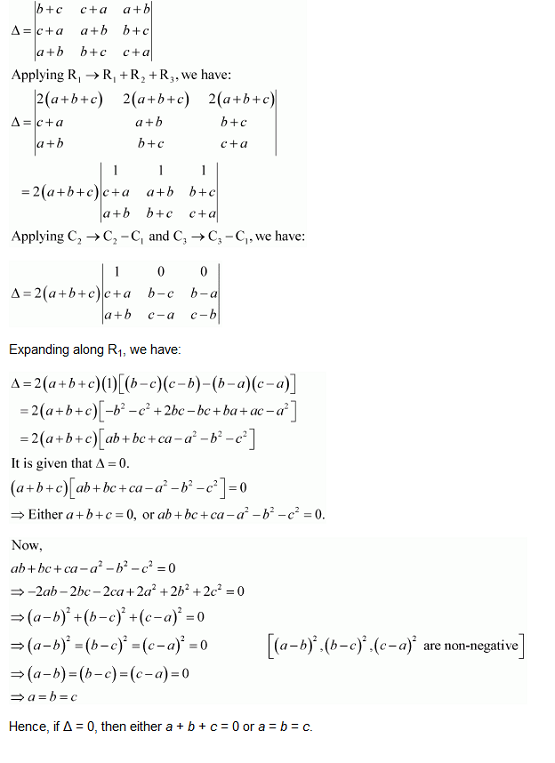Question5. Solve the equation: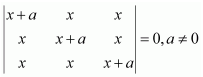Solution :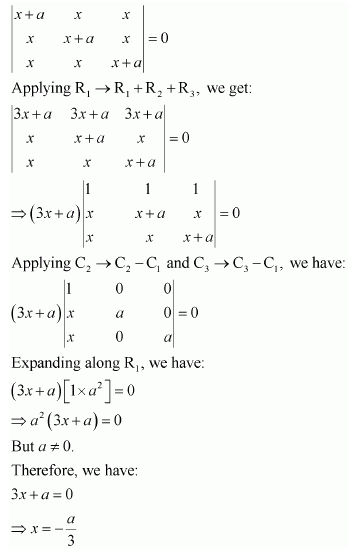Question6. Prove that: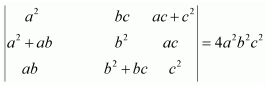Solution :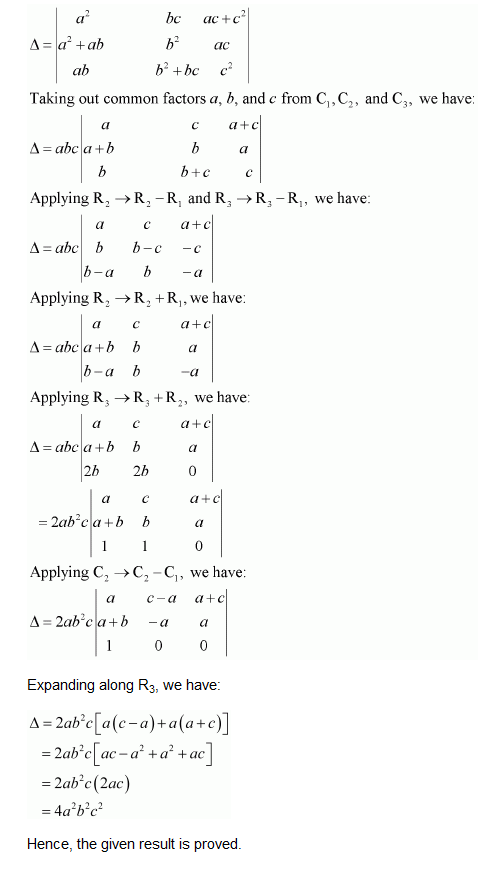Question7. If

Solution :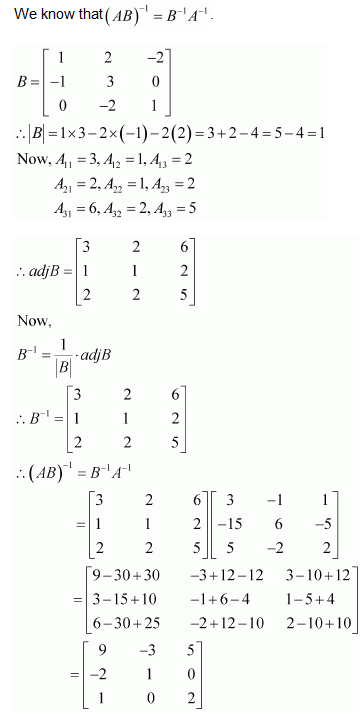Question8.

Solution :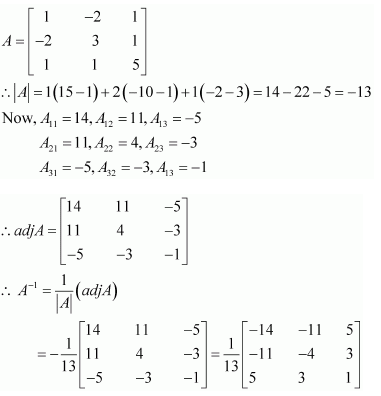Question9. Evaluate:

Solution :

Question10. Evaluate: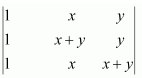Solution :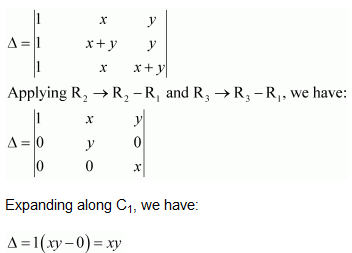Using properties of determinants in Exercises 11 to 15, prove that:

Question11.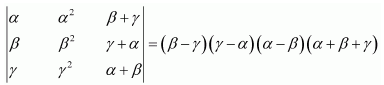Solution :

Question12.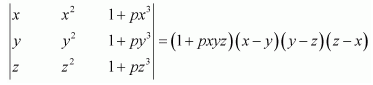Solution :

Question13.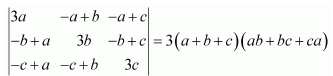Solution :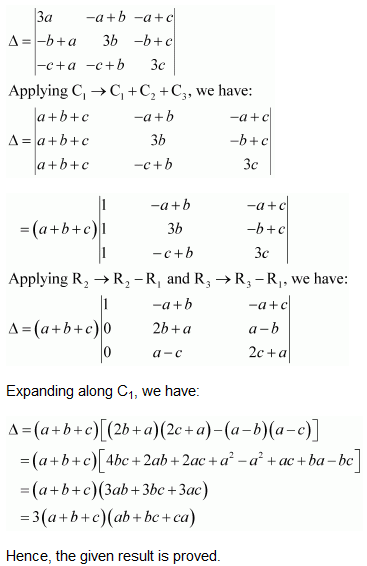Question14.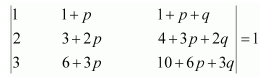Solution :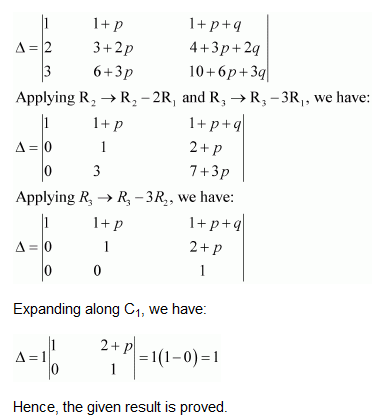Question15.

Solution :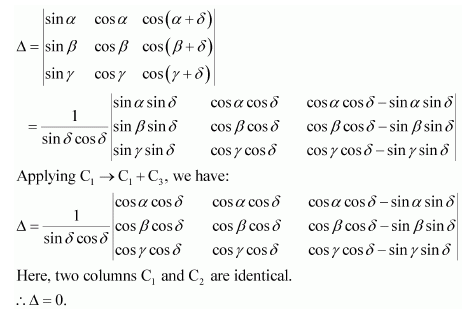Question16. Solve the system of the following equations: (Using matrices):

Solution :

Choose the correct answer in Exercise 17 to 19.

Question17. If a,b,c are in A.P., then the determinant is:

(A) 0

(B) 1

(C) x

(D) 2x

Solution :

Here, all the elements of the first row (R1) are zero.

Hence, we have Δ = 0.

Question18. If x,y,z are non-zero real numbers, then the inverse of matrix A =  is:

(A)

(B)

(C)

(D)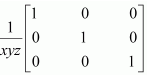Solution :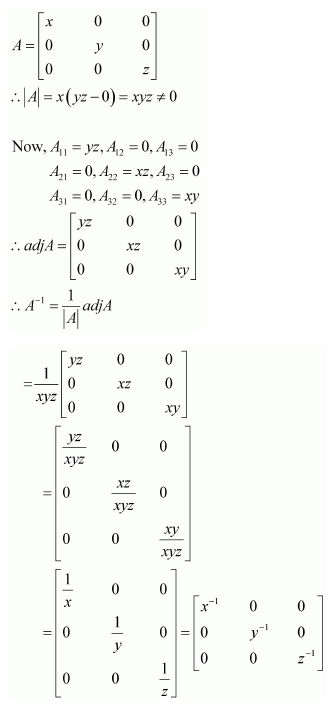Therefore, option (A) is correct.

Question19. Let where 0 ≤ θ≤ 2π, Then:

(A) Det (A) = 0

B. Det (A) ∈ (2, ∞)

C. Det (A) ∈ (2, 4)

D. Det (A)∈ [2, 4]

Solution :

Therefore, option (D) is correct.

Get 30% off your first purchase!

X
error: Content is protected !!
Scroll to Top